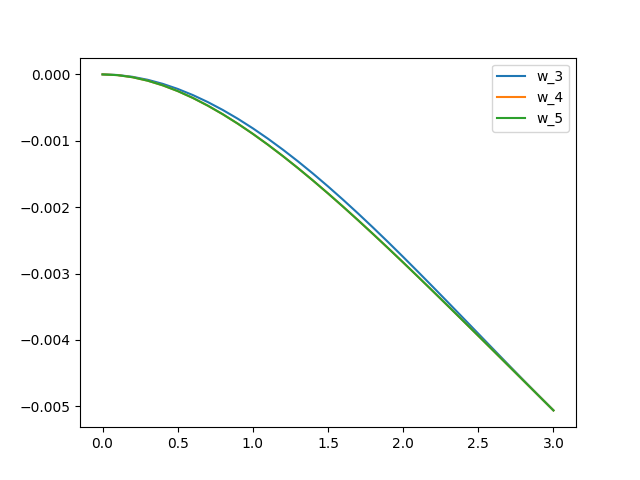# 1 Rayleigh-Ritz法的基本概念和求解步骤

## 1.1 弹性力学中的最小势能原理

$$\Pi \left(u_{i}\right)=U-W=\frac{1}{2} \int_{\Omega } \sigma_{ij} \epsilon_{ij} d\Omega -\int_{\Omega } \bar{p}_{i} u_{i}d\varGamma$$

$$\int_{\Omega } \left| \frac{\partial \Pi }{\partial u_{i}} \right| d\Omega =0$$

## 1.2 Rayleigh-Ritz法的求解步骤

1. 根据边界条件（Boundary Condition）建立位移形函数的表达式；
1. 弹性力学中的常用边界条件有两种：Essential Boundary Condition (EBC, 位移边界条件)，Natural Boundary Condition （NBC，受力边界条件），在Rayleigh-Ritz法中需要保证定义的位移形函数满足EBC条件；
2. 位移形函数一般选用完备函数的级数，如多项式级数、三角函数级数、小波技术、傅立叶级数等，对于二维变形三维变形等情况同样适用；
3. 若使用位移形函数描述二维问题时，如悬臂梁、简支梁等，则在定义位移形函数时需要让位移形函数满足体系的边界条件，如悬臂梁在在$x=0$处的位移为0，则悬臂梁的变形曲线可以描述为：$w(x)=\sum^{n}_{i=1} a_{i}x^{i}$，保证悬臂梁的固定处满足$w(0)=0$
4. 若使用位移形函数描述二维甚至多维问题时，如板的变形，体的变形，同样需要满足边界条件，且在Rayleigh-Ritz法的计算过程中必须保证定义的边界条件为EBC；
2. 计算弹性体系在当前的位移形函数下的应变能；

1. 对于二维问题，一般应用Rayleigh-Ritz法计算弯曲变形梁的应变能，需要根据梁的厚高比选取合适的梁模型，常用的梁模型包括Euler-Bernoulli梁和Timoshenko梁模型，主要区别在于Timoshenko梁模型考虑梁的剪切应变能；
2. Euler-Bernoulli梁模型的应变能表达式为$U_E=\frac{EI}{2}\int_0^L (\frac{d^2u}{dx^2})dx$;
3. Timoshenko梁模型的应变能表达式为$U_T=\frac{EI}{2}\int_0^L{(\frac{d^2u}{dx^2})dx} + \frac{\alpha(EI)^2}{2GA}$，其中$\alpha_s$为不均匀剪切系数，$\alpha_s = \frac{A}{I^2}\int_A \frac{S^2}{b^2}dA$，其与截面形式有关，一般矩形截面取为$\alpha_s = \frac{6}{5}$
4. 对于三维问题，一般应用Rayleigh-Ritz法计算板的应变能，需要根据板厚度和两个方向的尺度比选取合适的板模型，比较常用的是高阶剪切板理论，这里介绍一下我副导师文章中提出的（General Third-Shear Deformation Plate Theory）
5. 剪切板理论的变形能计算方法：

$$U=\frac{EI}{2} \int_{\Omega }{ \left( \frac{\partial^{2} w}{\partial x^{2}} +\frac{\partial^{2} w}{\partial y^{2}} \right) -2\left( 1-\nu \right) \left( \frac{\partial^{2} w}{\partial x^{2}} \frac{\partial^{2} w}{\partial y^{2}} -\left( \frac{\partial^{2} w}{\partial x\partial y} \right)^{2} \right) dxdy} + U_s$$

1. 其中$U_s$是考虑剪切效应的剪切应变能。
2. 当所选用的剪切板模型GTSDPT时，剪切应变能的计算表达式为：
$$U_{s}=G\int_{\Omega }{ \left( \frac{\partial u}{\partial y} +\frac{\partial v}{\partial x} +z\frac{\partial \theta }{\partial y} +z\frac{\partial \varphi }{\partial x} \right) +\left( \theta +\frac{\partial w}{\partial x} \right)^{2} +\left( \varphi +\frac{\partial w}{\partial y} \right)^{2} d\Omega }$$
3. 计算弹性体系受到的外力做功，若弹性体的变形与外力的变形一致，则外力做正功，否则做负功。弹性体系的变形能按照总势能表达式的中的方程进行计算； 4. 计算获得总势能表达式后，假定位移形函数中的待定系数的集合为${a_i}$，对弹性体系总势能计算关于位移形函数的关于待定系数的变分：
$$\frac{\partial \Pi }{\partial a_{i}} =0$$
5. 求解代数方程组，获得待定系数，将待定系数代入至位移形函数中，获得弹性体系的位移函数。 ## 1.3 算例 如图所示是一个悬臂梁模型，梁的长度为$L=3m$，一端固定在墙上，一端自由。梁上分布了$2kN/m$的均布荷载，计算梁的挠度函数。 ![算例几何模型](example1.png) ### 1.3.1 材料力学解法 在材料力学中，基于弹性、各向同性、连续性、小变形等假定，在欧拉梁模型下，梁的挠度与受力关系满足以下基本假定：
$$\frac{d^2w(x)}{x} = - \frac{M(x)}{EI}$$

$$F_s(x) = 30 - 10x$$

$$M(x) = \int_0^x 30 - 10x dx\\ = 30x - 5x^2 + C \\$$

$$M(3) = 90 - 45 + C = 0 \\ => C = -45 \\ M(x) = 30x - 5x^2 - 45$$

$$\theta(x) = \frac{dw}{dx} = -\frac{\int_0^x 30x - 5x^2 - 45dx}{EI} \\ =\frac{15x^2 - \frac{5}{3} x^3 - 45x + C}{EI} \\ \theta(0) = -\frac{C}{EI} = 0 \\ => \theta(x) = -\frac{15x^2 - \frac{5}{3} x^3 - 45x}{EI}$$

$$w(x)=\int_0^x \theta(x)dx=-\frac{5x^3-\frac{5}{12}x^4-\frac{45}{2}x^2}{EI} \\ =-\frac{5x^3-\frac{5}{12}x^4-\frac{45}{2}x^2}{2E4}$$

$$w(3) = 0.0050625m = 5.0625 mm$$

### 1.3.2 Rayleigh-Ritz法解法

$$w(x) = a_0x^2 + a_1x^3 + a_2x^4$$

$$U = 120000.0 a_{0}^{2} + 1080000.0 a_{0} a_{1} + 4320000.0 a_{0} a_{2} + 3240000.0 a_{1}^{2} + 29160000.0 a_{1} a_{2} + 69984000.0 a_{2}^{2}$$

$$W = \int_0^3 10w(x) dx \\ = 90 a_{0} + \frac{405 a_{1}}{2} + 486 a_{2}$$

$$\Pi = U - W \\ = 120000.0 a_{0}^{2} + 1080000.0 a_{0} a_{1} + 4320000.0 a_{0} a_{2} - 90 a_{0} + 3240000.0 a_{1}^{2} + 29160000.0 a_{1} a_{2} - \frac{405 a_{1}}{2} + 69984000.0 a_{2}^{2} - 486 a_{2}$$

$$\frac{\partial \Pi}{\partial a_i} = 0$$

$$240000.0 a_{0} + 1080000.0 a_{1} + 4320000.0 a_{2} - 90=0$$ $$1080000.0 a_{0} + 6480000.0 a_{1} + 29160000.0 a_{2} - \frac{405}{2}=0$$ $$4320000.0 a_{0} + 29160000.0 a_{1} + 139968000.0 a_{2} - 486=0$$ 
求解方程组，得到挠度函数的表达式：
$$w(x) = 2.08333333333333 \cdot 10^{-5} x^{4} - 0.00025 x^{3} + 0.001125 x^{2}$$

### 1.3.3 灵敏度分析

$$w_3(x) = - 0.000125 x^{3} + 0.0009375 x^{2} \\ w_4(x) = 2.08333333333333 \cdot 10^{-5} x^{4} - 0.00025 x^{3} + 0.001125 x^{2}\\ w_5(x) = 2.08333333333333 \cdot 10^{-5} x^{4} - 0.00025 x^{3} + 0.001125 x^{2}$$https://www.eatrice.cn/post/RayleighRitzMethod/

2023年7月9日